Example Questions

← Previous 1 3 4 5

Example Question #1 : How To Find The Radius Of A Sphere

A cube with volume 27 cubic inches is inscribed inside a sphere such that each vertex of the cube touches the sphere. What is the radius, in inches, of the sphere?

8.5

√3/2 (approximately 1.73)

(3√3)/2 (approximately 2.60)

9

(3√3)/2 (approximately 2.60)

Explanation:

We know that the cube has a volume of 27 cubic inches, so each side of the cube must be ∛27=3 inches. Since the cube is inscribed inside the sphere, the diameter of the sphere is the diagonal length of the cube, so the radius of the sphere is half of the diagonal length of the cube. To find the diagonal length of the cube, we use the distance formula d=√(32+32+32 )=√(3*32 )=3√3, and then divide the result by 2 to find the radius of the sphere, (3√3)/2.

Example Question #2 : How To Find The Radius Of A Sphere

The surface area of a sphere is 100π square feet.  What is the radius in feet?

100

25

π

10

5

5

Explanation:

S = 4π(r2)

100π = 4π(r2)

100 = 4r2

25 = r2

5 = r

Example Question #3 : How To Find The Radius Of A Sphere

What is the radius of a sphere with a surface area of 16?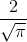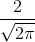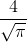Explanation:

In order to find the radius, first write the surface area formula for a sphere and substitute the surface area.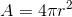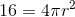Divide byon both sides in order to isolate the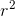term.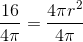Simplify both sides of the equation.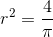Square root both sides.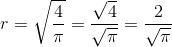The radius is.

Example Question #1 : Spheres

Find the diameter of a sphere with a surface area of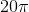.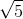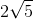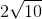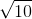Explanation:

Write the formula to find the surface area of a sphere.Substitute the area and solve for the radius.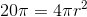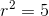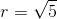The diameter is double the radius.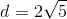Example Question #2 : Spheres

What is the diameter of a sphere if the surface area is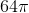?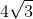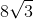Explanation:

Write the formula for the surface area of a sphere.Substitute the area and find the radius.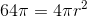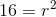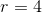The diameter is double the radius.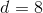Example Question #3 : Spheres

What is the diameter of a sphere with a volume of?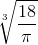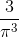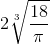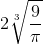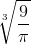Explanation:

Write the formula for the volume of a sphere.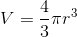Substitute the volume.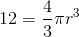Multiply by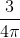on both sides in order to isolate the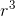term.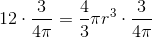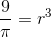Cube root both sides.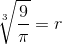The diameter is double the radius.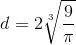The answer is:Example Question #1 : How To Find The Surface Area Of A Sphere

A spherical orange fits snugly inside a small cubical box such that each of the six walls of the box just barely touches the surface of the orange.  If the volume of the box is 64 cubic inches, what is the surface area of the orange in square inches?

32π

64π

16π

128π

256π

16π

Explanation:

The volume of a cube is found by V = s3.  Since V = 64, s = 4.  The side of the cube is the same as the diameter of the sphere.  Since d = 4, r = 2.  The surface area of a sphere is found by SA = 4π(r2) = 4π(22) = 16π.

Example Question #2 : How To Find The Surface Area Of A Sphere

A solid sphere is cut in half to form two solid hemispheres. What is the ratio of the surface area of one of the hemispheres to the surface area of the entire sphere before it was cut?

3/4

1/2

3/2

2/3

1

3/4

Explanation:

The surface area of the sphere before it was cut is equal to the following:

surface area of solid sphere = 4πr2, where r is the length of the radius.

Each hemisphere will have the following shape:In order to determine the surface area of the hemisphere, we must find the surface area of the flat region and the curved region. The flat region will have a surface area equal to the area of a circle with radius r.

area of flat part of hemisphere = πr2

The surface area of the curved portion of the hemisphere will equal one-half of the surface area of the uncut sphere, which we established to be 4πr2.

area of curved part of hemisphere = (1/2)4πr= 2πr2

The total surface area of the hemisphere will be equal to the sum of the surface areas of the flat part and curved part of the hemisphere.

total surface area of hemisphere = πr+ 2πr= 3πr2

Finally, we must find the ratio of the surface area of the hemisphere to the surface area of the uncut sphere.

ratio = (3πr2)/(4πr2) = 3/4

Example Question #3 : How To Find The Surface Area Of A Sphere

The volume of a sphere is 2304π in3.  What is the surface area of this sphere in square inches?

576π

144π

216π

36π

576π

Explanation:

To solve this, we must first begin by finding the radius of the sphere. To do this, recall that the volume of a sphere is:

V = (4/3)πr3

For our data, we can say:

2304π = (4/3)πr3; 2304 = (4/3)r3; 6912 = 4r3; 1728 = r3; 12 * 12 * 12 = r3; r = 12

Now, based on this, we can ascertain the surface area using the equation:

A = 4πr2

For our data, this is:

A = 4π*122 = 576π

Example Question #4 : How To Find The Surface Area Of A Sphere

A sphere has its center at the origin.  A point on its surface is found on the x-y axis at (6,8).  In square units, what is the surface area of this sphere?

40π

200π

400π

(400/3)π

400π

Explanation:

To find the surface area, we must first find the radius.  Based on our description, this passes from (0,0) to (6,8).  This can be found using the distance formula:

62 + 82 = r2; r2 = 36 + 64; r2 = 100; r = 10

It should be noted that you could have quickly figured this out by seeing that (6,8) is the hypotenuse of a 6-8-10 triangle (which is a multiple of the "easy" 3-4-5).

The rest is easy.  The surface area of the sphere is defined by:

A = 4πr2 = 4 * 100 * π = 400π

← Previous 1 3 4 5